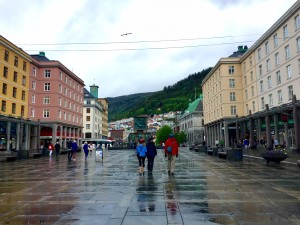# Traversing Mountain PassesHow can you (mathematically) avoid walking in deep puddles? Photo by Alexi Hoeft, used with permission.

Suppose you need to walk through a wet parking lot. The lot is covered with puddles and you would like to keep your shoes as dry as possible. If you know the depth of the puddles at every point, how do you choose the path that minimizes the maximum depth of the puddles you cross? A hiker might want to solve a similar problem if they want to avoid fatigue by seeking low elevations. How do you traverse a mountainous area while remaining as low as possible?

To make the problem more formal, say the area of interest is$X=[0,1]\times[0,1]$ and the elevation of the ground (or puddle depth) at each point$(x,y) \in X$ is$f(x,y)$, with$f:X\to\mathbb{R}$ continuous. Choose areas that are acceptable start and finish points and call them$X_0$ and$X_1$. If you want to walk from the north end of the square to the south, then you could choose$X_0=[0,1]\times\{1\}$ and$X_1=[0,1]\times\{0\}$. The problem can be stated as the minimax$\displaystyle{\min_P \max_t f(P(t))}$

over continuous paths$P:[0,1]\to X$ such that$P(0)\in X_0$ and$P(1)\in X_1$.

Initially, you might suppose that this is a fundamentally continuous problem since there is a continuum of possible routes$P$. However, we can make the problem discrete by considering sublevel sets of$f$.

The$c$-sublevel set of$f$ is the set of points where$f$ is below elevation$c$, that is$S_c=\{(x,y)\in X | f(x,y)\leq c\}$.

The minimax problem is solved by finding a$P$ that lies in the lowest sublevel set connecting$X_0$ and$X_1$. That lowest elevation is$\min\{c | \exists P: P(0)\in X_0,\, P(1)\in X_1 \text{ and } \forall t\in[0,1]: P(t)\in S_c\}$.

Watch the animated figure below and notice the first time that the white sublevel set connects the top and bottom. A path between top and bottom must first appear when the boundaries pass through a saddle point of$f$ because saddle points are where connected components of sublevel sets join each other. This is a consequence of the “mountain pass theorem”: if$P^*$ is optimal and$f(P^*(t))$ is maximized by$t^*$, then$P^*(t^*)$ is a stationary point of$f$. That stationary point is a normally a saddle point (though it could be part of a flat-topped area).Now we can write a discrete process for finding an optimal path:

1. Locate all saddle points of$f$.
2. Order the saddle points$\{(x_1,y_1),\dots,(x_n,y_n)\}$ by elevation so that$f(x_1,y_1) \leq \cdots \leq f(x_n,y_n)$.
3. For each saddle point$(x_i,y_i)$, determine whether its sublevel set$S_{f(x_i,y_i)}$ has a connected component intersecting$X_0$ and$X_1$.
4. If the lowest such saddle point is$(x_{\hat i},y_{\hat i})$, then$f(x_{\hat i},y_{\hat i})$ is the minimum value for the minimax problem, and the optimal path is any$P$ contained in$S_{f(x_{\hat i},y_{\hat i})}$ that connects$X_0$ and$X_1$.

For something else to think about, let’s consider a connection to graph theory. If you watch the above animation a few times, you’ll notice that connected components of the white sublevel sets are created at local minima. They spread out and meet other components at saddle points. You might imagine a graph (or network) being created between the local minima: when two components touch, an edge is created between two minima (one in each component).

Is there a natural way to choose a representative from each component to connect? If a hole forms so that the component is no longer simply connected, should an edge be added to make a cycle? Also, a graph on the maxima could be created in the same way. Should the graph on the maxima be the dual of the graph on the minima?

Post any thoughts or questions in the comments below!